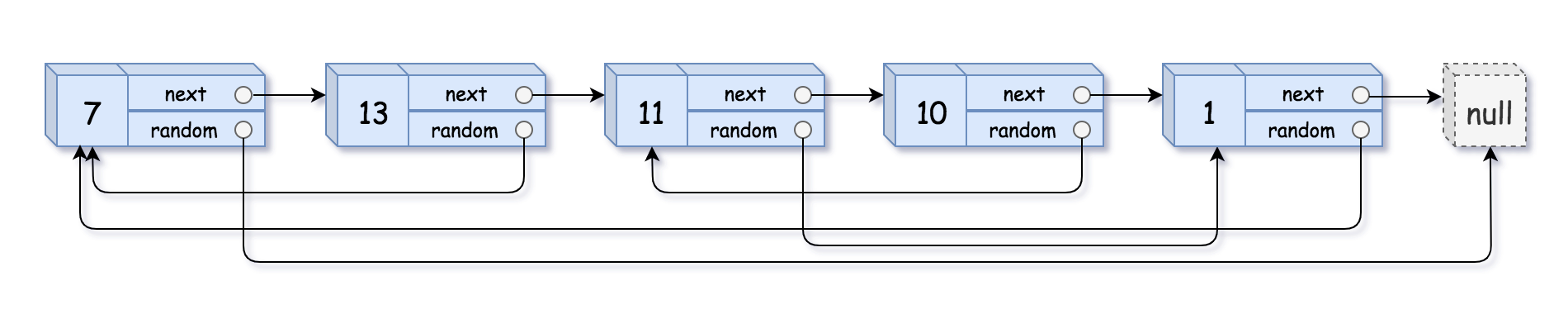0138. 复制带随机指针的链表

# 0138. 复制带随机指针的链表#

• 标签：链表、哈希表
• 难度：中等

## 题目大意 #

• $0 \le n \le 1000$。
• $-10^4 \le Node.val \le 10^4$。
• Node.randomnull 或指向链表中的节点。1 2  输入：head = [[7,null],[13,0],[11,4],[10,2],[1,0]] 输出：[[7,null],[13,0],[11,4],[10,2],[1,0]]1 2  输入：head = [[1,1],[2,1]] 输出：[[1,1],[2,1]] 

## 解题思路 #

### 思路 1：迭代 #

1. 遍历链表，利用哈希表，以 旧节点: 新节点 为映射关系，将节点关系存储下来。
2. 再次遍历链表，将新链表的 nextrandom 指针设置好。

### 思路 1：代码 #

  1 2 3 4 5 6 7 8 9 10 11 12 13 14 15 16 17 18  class Solution: def copyRandomList(self, head: 'Node') -> 'Node': if not head: return None node_dict = dict() curr = head while curr: new_node = Node(curr.val, None, None) node_dict[curr] = new_node curr = curr.next curr = head while curr: if curr.next: node_dict[curr].next = node_dict[curr.next] if curr.random: node_dict[curr].random = node_dict[curr.random] curr = curr.next return node_dict[head] 

### 思路 1：复杂度分析 #

• 时间复杂度：$O(n)$。
• 空间复杂度：$O(n)$。